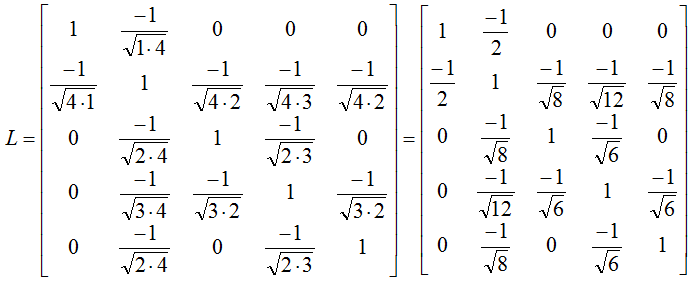Engineering Math - Quick Reference                                 Home : www.sharetechnote.com Graph Theory - Laplacian/Combinatorial Laplacian/Normalized Laplacian   Formal definition of Laplacian is as follows.  In a Graph G, Laplacian L is defined as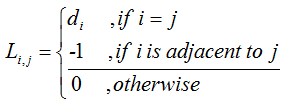What is 'di' ? It is the diagonal elelment of 'Degree Matrix'. Actually, Laplacian can be obtained by combining the degree matrix and the adjacency matrix as follows.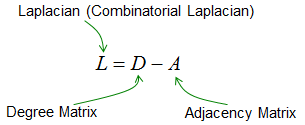Example >   Let's assume that we have a Graph as shown below.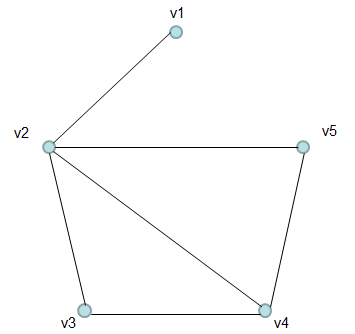The adjacency matrix of this graph is as follows (try to build this matrix on your own as a practice).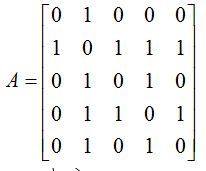The degree matrix of this graph is as follows (try to build this matrix on your own as a practice).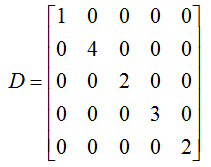The Laplacian of this graph calculated from 'D-A' become as follows.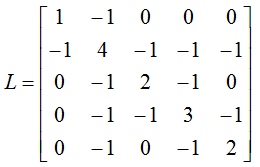In many case, 'Normalized Laplacian' are used. The Normalized Laplacian is defined as follows. (As you see, we divide all the elements of Laplacian in such a way that the diagonal values become '1'. (di is the diagonal values on Laplacian matrix).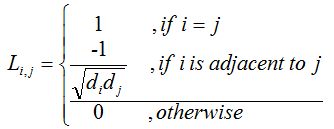Normalized Laplacian given in this example become as follows :